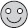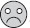# User Forum

Subject :IMO    Class : Class 3

48 ÷=. Ifis an even number andis also an even number, what could not be these two numbers?

A= 2,= 24
B= 3,= 16
C= 4,= 12
D= 6,= 8

how can this smiley be an odd number when it is asked even

## Ans 1:

Class : Class 5
The answer can be 48\6=8 also

Class : Class 3
b